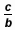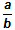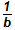# Activities

••• ##### Subject Area

• Math: Elementary Math: Fractions

• ##### AuthorK-5

30 Minutes

• ##### Device
•TI-Nspire™ CX/CX II
•TI-Nspire™ CX CAS/CX II CAS
• ##### Software

TI-Nspire™
TI-Nspire™ CAS

## Adding and Subtracting Fractions with Common Denominators

#### Activity Overview

This activity uses interactive number lines to investigate addition and subtraction of fractions that refer to the same whole, with like denominators. Students may reason about the process as 1) decomposing each fraction into unit fractions and counting the total number of unit fractions that would be marked on the number line; or 2) the series of the lengths from 0 represented by each fraction, when those segments are joined end to end.

This TI-Nspire lesson uses interactive number lines to investigate addition and subtraction of fractions that refer to the same whole, with like denominators. Students may reason about the process as 1) decomposing each fraction into unit fractions and counting the total number of unit fractions that would be marked on the number line; or 2) the series of the lengths from 0 represented by each fraction, when those segments are joined end to end. Subtraction can be thought of as finding the missing addend (i.e. for-, what would you add toto obtain?) or as taking away the number of copies offrom the number of copies of.
Students should be familiar with the concept of fraction as a point on a number line before they begin this activity. Understandingas a copies ofmarked on the number line beginning from 0 suggests that adding two fractions with denominators ofis the same as joining the first segmentend to end with the second segment. The activity can also be used to investigate the properties for addition (commutative, associative, inverse, and identity).